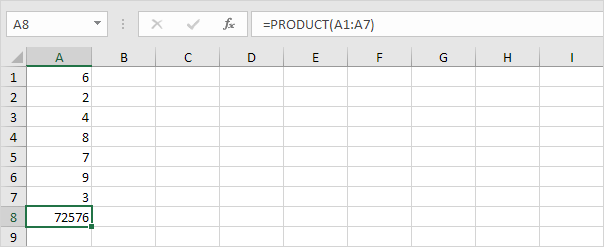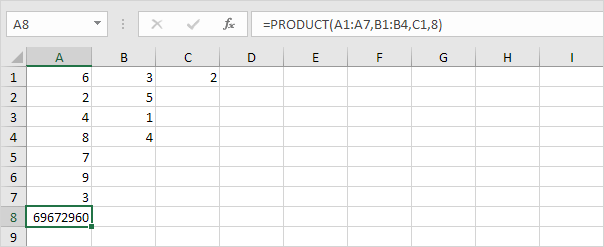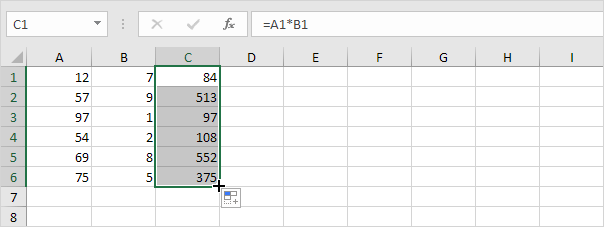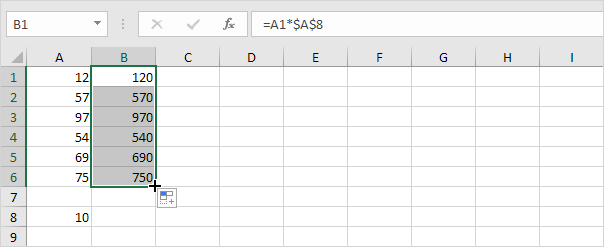# Multiply

To multiply numbers in Excel, use the asterisk symbol (*) or the PRODUCT function. Learn how to multiply columns and how to multiply a column by a constant.

1. The formula below multiplies numbers in a cell. Simply use the asterisk symbol (*) as the multiplication operator. Don’t forget, always start a formula with an equal sign (=).2. The formula below multiplies the values in cells A1, A2 and A3.3. As you can imagine, this formula can get quite long. Use the PRODUCT function to shorten your formula. For example, the PRODUCT function below multiplies the values in the range A1:A7.4. Here’s another example.Explanation: =A1*A2*A3*A4*A5*A6*A7*B1*B2*B3*B4*C1*8 produces the exact same result.

Take a look at the screenshot below. To multiply two columns together, execute the following steps.

5a. First, multiply the value in cell A1 by the value in cell B1.5b. Next, select cell C1, click on the lower right corner of cell C1 and drag it down to cell C6.Take a look at the screenshot below. To multiply a column of numbers by a constant number, execute the following steps.

6a. First, multiply the value in cell A1 by the value in cell A8. Fix the reference to cell A8 by placing a \$ symbol in front of the column letter and row number (\$A\$8).6b. Next, select cell B1, click on the lower right corner of cell B1 and drag it down to cell B6.Explanation: when we drag the formula down, the absolute reference (\$A\$8) stays the same, while the relative reference (A1) changes to A2, A3, A4, etc.

If you’re not a formula hero, use Paste Special to multiply in Excel without using formulas!

7. For example, select cell C1.8. Right click, and then click Copy (or press CTRL + c).

9. Select the range A1:A6.

10. Right click, and then click Paste Special.

11. Click Multiply.12. Click OK.Note: to multiply numbers in one column by numbers in another column, at step 7, simply select a range instead of a cell.

Previous articleLocate Maximum Value in Excel
Next articlePercentage Formula in Excel – Easy Calculations